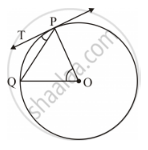Share

# In Figure 1, O is the Centre of a Circle, Pq is a Chord and Pt is the Tangent at P. If ∠Poq = 70°, Then ∠Tpq is Equal to - Mathematics

Course
ConceptCircles Examples and Solutions

#### Question

In figure 1, O is the centre of a circle, PQ is a chord and PT is the tangent at P.

If ∠POQ = 70°, then ∠TPQ is equal to• A. 55°

• B. 70°

• C. 45°

• D. 35°

#### Solution

In ΔOPQ:

OP = OQ (Radii of same circle)

⇒ ∠OQP = ∠OPQ (Equal sides have equal angles opposite to them)

∠POQ + ∠OPQ + ∠OQP = 180° (Angle sum property of triangles)

⇒ 70° + 2∠OPQ = 180°

⇒ 2∠OPQ = 180° − 70° = 110°

⇒ ∠OPQ = 55°

It is known that the tangent is perpendicular to the radius through the point of contact.

∴ ∠OPT = 90°

⇒ ∠OPQ + ∠TPQ = 90°

⇒ 55° + ∠TPQ = 90°

⇒ ∠TPQ = 90° − 55° = 35°